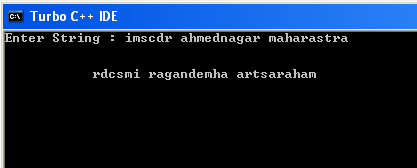## Translate

### C Program example to reverse each world of given sentence

C Program to reverse each world of given sentence solve C Program to reverse each world of given sentence with source code, how to write c program to revers each world

#include
#include
void main()
{
char string,choice;
int i=0,j=0,k=0;
char temp;
clrscr();

printf("Enter String :");
gets(string);
printf("\n \n \t ") ;
while (string[i]!='\0')
{
//here we find spaces from string and store index of space in j
if (string[i]==' ')
{
j=i;
while(k<=i)
{
temp[k]=string[j--];
printf("%c",temp[k]);
k++;
}
}
i++;
}
//this is for word after space occur in string
if (string[i]=='\0')
{
j=i;
while(k<=i){
temp[k]=string[j--];
printf("%c",temp[k]);
k++;
}

}
getch();
}